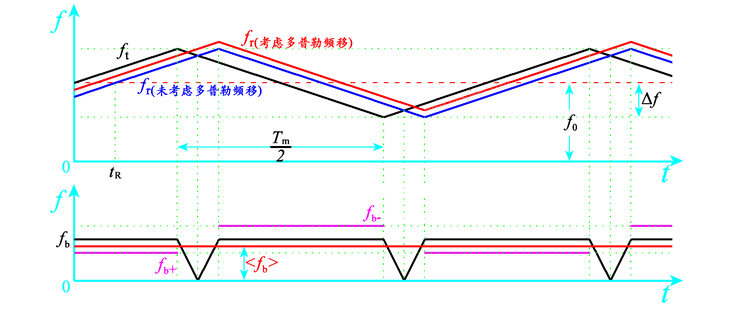### 原理$$f _m = \frac{1}{T _m}$$，是三角调频的频率(不是发射信号的频率)。

### 运动目标

$f _{b+} = f _t - f _r = \frac{8\Delta f R}{T _m c} - f _d$ $f _{b-} = f _t - f _r = \frac{8\Delta f R}{T _m c} + f _d$

### 误差

$$\frac{\Delta f _b}{f _m}$$表示在一个调制周期 $$\frac{1}{f _m}$$内差频的误差，当频率测读量化误差为1次，即$$\frac{\Delta f _b}{f _m}=1$$时，有